Courses

# Capacitors - Physics, Solution by DC Pandey NEET Notes | EduRev

## DC Pandey (Questions & Solutions) of Physics: NEET

Created by: Ciel Knowledge

## NEET : Capacitors - Physics, Solution by DC Pandey NEET Notes | EduRev

The document Capacitors - Physics, Solution by DC Pandey NEET Notes | EduRev is a part of the NEET Course DC Pandey (Questions & Solutions) of Physics: NEET.
All you need of NEET at this link: NEET

Introductory Exercise 22.1

Ques 1: Find the dimensions of capacitance.
Sol: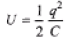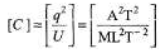= [M-1L-2T4A2]

Ques 2: No charge will flow when two conductors having the same charge are connected to each other. Is this statement true or false?
Sol:
Charge does not flow if their potentials are same.

Ques 3: Two metallic plates are kept parallel to one another and charges are given to them as shown in figure. Find the charge on all the four faces.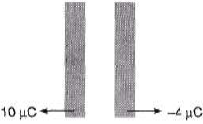Sol:
Charge on outermost surfaces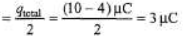Hence, charges are as shown below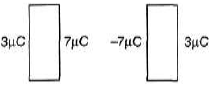Ques 4: Charges 2q and -3g are given to two identical metal plates of area of cross-section A. The distance between the plates is d. Find the capacitance and potential difference between the plates.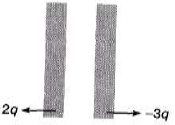Sol:
Charge on outermost surfaces.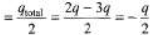Hence charge on different faces are as shown below.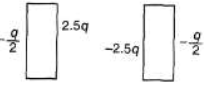Electric field and hence potential difference between the two plates is due to ±2.5 q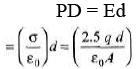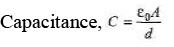Introductory Exercise 22.2

Ques 1: Find the charge stored in all the capacitors.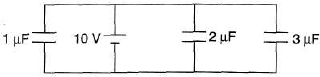Sol:
All three capacitors are in parallel wit h the battery. PD across each of them is 10V. So, apply q = CV for all of them.

Ques 2: Find the charge stored in the capacitor.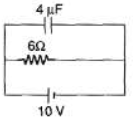Sol:
Capacitor and resistor both are in parallel wit h the battery. PD across capacitor is 10V. Now apply q = CV.

Ques 3: Find the charge stored in the capacitor.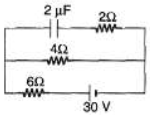Sol:
In steady state current flows in lower loop of the circuit.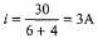Now, potential difference across capacitor = potential difference across 4Ω resistance.
= iR
= (3)(4)=12V
q = CV = (2μF) (12 V)
= 24μC

Ques 4: A 2μF capacitor and a 2μF capacitor are connected in series across a 1200 V supply line.
(a) Find the charge on each capacitor and the voltage across them.
(b) The charged capacitors are disconnected from the line and from each other and reconnected with terminals of like sign together. Find the final charge on each and the voltage across them.

Sol: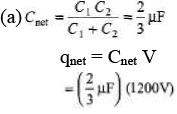= 800μC
In series, q remains same
q1 = q2 = 800 μC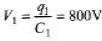and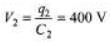(b) Now total charge will become 1600 μC. This will now distribute in direct ratio of capacity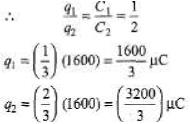They will have a common potential (in parallel) given by.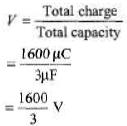Ques 5: A 100 μF capacitor is charged to 100 V. After the charging, battery is disconnected. The capacitor is then connected in parallel to another capacitor. The final voltage is 20 V. Calculate the capacity of second capacitor.
Sol:
Charge, q = CV = 104μC
In parallel common potential is given by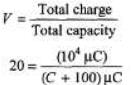Solving this equation we get
C = 400μF

Introductory Exercise 22.3

Ques 1: An uncharged capacitor C is connected to a battery through a resistance R. Show that by the time the capacitor gets fully charged, the energy dissipated in R is the same as the energy stored in C.
Sol:
Charge supplied by the battery,
q= CV
Energy supplied by the battery,
E = qV =CV2
Energy stored in the capacitor,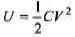∴ Energy dissipated across R in the form of heat = E - U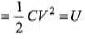Ques 2: How many time constants will elapse before the current in a charging RC circuit drops to half of its initial value?
Sol: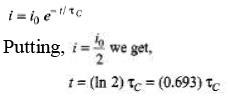Ques 3: A capacitor of capacitance C is given a charge q0. At time t = 0 it is connected to an uncharged capacitor of equal capacitance through a resistance R. Find the charge on the first capacitor and the second capacitor as a function of time f. Also plot the corresponding q-t graphs.
Sol:
Both capacitors have equal capacitance. Hence half-half charge distribute over both the capacitors.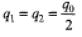q1 decreases exponentially from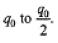While q2 increases exponentially from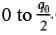Corresponding graphs and equation are given in the answer. Time constant of two exponential equations will be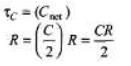Ques 4: A capacitor of capacitance C is given a charge q0. At time f = 0, it is connected to a battery of emf E through a resistance R. Find the charge on the capacitor at time t.
Sol: qi = q0 qf = EC
Now charge on capacitor changes from qto qf exponentially.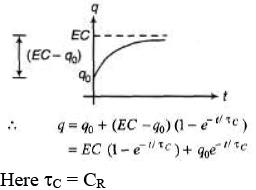Ques 5: Determine the current through the battery in the circuit shown in figure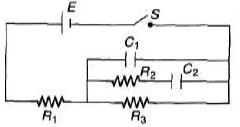(a) immediately after the switch S is closed
(b) after a long time.
Sol: (a) Immediately after the switch is closed whole current passes through C
i = E/R1
(b) Long after switch is closed no current will pass through C1 and C2.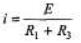Ques 6: For the circuit shown in figure find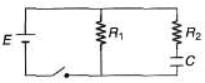(a) the initial current through each resistor
(b) steady state current through each resistor
(c) final energy stored in the capacitor
(d) time constant of the circuit when switch is opened.
Sol:
(a) At t = 0 equivalent resistance of capacitor is zero. R1 and R2 are in parallel across the battery PD across each is E.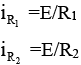(b) In steady state no current flow through capacitor wire. PD across R1 is E.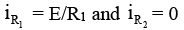(c) In steady state potential difference across capacitor is E.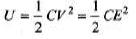(d) When switch is opened, capacitor is discharged through resistors R1 and R2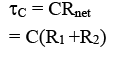Offer running on EduRev: Apply code STAYHOME200 to get INR 200 off on our premium plan EduRev Infinity!

210 docs

,

,

,

,

,

,

,

,

,

,

,

,

,

,

,

,

,

,

,

,

,

,

,

,

;